# Mathematical Modelling for Heat Transfer of a Micropolar Fluid along a Permeable Stretching/Shrinking Wedge with Heat Generation/Absorption

Mathematical Modelling for Heat Transfer of a Micropolar Fluid along a Permeable Stretching/Shrinking Wedge with Heat Generation/Absorption

M.S. AlamTarikul Islam M.J. Uddin

Department of Mathematics, Jagannath University, Dhaka-1100, Bangladesh

Department of Mathematics and Statistics, Bangladesh University of Business and Technology, Dhaka-1216, Bangladesh

Department of Mathematics and Statistics, Sultan Qaboos University, PO Box 36, PC 123 Al-Khod, Muscat, Sultanate of Oman

Corresponding Author Email:
dralamjnu@gmail.com
Page:
1-9
|
DOI:
https://doi.org/10.18280/mmep.030101
N/A
|
Accepted:
N/A
|
Published:
31 March 2016
| Citation

OPEN ACCESS

Abstract:

In this paper, we have developed a mathematical model for the problem of unsteady hydromagnetic two dimensional flow and heat transfer of a viscous incompressible micropolar fluid over a heated permeable linearly stretching/shrinking wedge with heat generation/absorption. A set of similarity parameters is employed to convert the governing nonlinear partial differential equations into ordinary differential equations which are solved numerically using Nachtsheim-Swigert shooting iteration technique along with sixth order Runge-Kutta integration scheme. Comparisons with previously published work are performed and the results are found to be in excellent agreement. Numerical results are presented through graphs and tables and discussed them from the physical point of view. It is observed that the local skin friction coefficient (rate of shear stress) increases with the increase of the magnetic field parameter and the rate of heat transfer increases with the increase of the heat generation parameter.

Keywords:

modelling, hydromagnetic, shrinking/stretching wedge, heat generation/absorption

1. Introduction

Micropolar fluids are referred to those fluids that contain micro-constituents that can undergo rotation which affect the hydrodynamics of the flow. The dynamics of micropolar fluids, originated from the theory of Eringen [1, 2] has been a popular area of research due to their applications in a number of processes that occurs in industry. The development of research in this area is stimulated by the presence of a variety of its real world applications in industrial and engineering processes. Exotic lubricants, colloidal suspensions, solidification of liquid crystals, extrusion of polymer fluids, cooling of metallic plate in bath, animal bloods, body fluids, glass fiber, glass blowing, paper production and rubber sheets are examples of these applications. Also, the study of heat generation/absorption in moving fluids is important in problems with chemical reactions and those concerned with dissociating fluids. Possible heat generation effects may alter the temperature distribution; consequently, the particle deposition rate in nuclear reactors, electronic chips and semi conductor wafers. The number of investigations in the convective flow and heat transfer over a stretching/shrinking wedge has grown dramatically in recent years. Crane  was the first to report the analytical solution for the boundary layer flow of an incompressible viscous fluid over a stretching plate. On the other hand, it seems that Miklavčič and Wang  were the first who investigated the flow over a shrinking sheet. After the pioneering contributions by both Crane  and Miklavčič and Wang , the study of fluid flow over a stretching/shrinking sheet has been explored by a large number of researchers under different physical conditions. An excellent review of micropolar fluids and their applications was given by Ariman et al. [5, 6]. Soundealgekar and Takhar  studied the flow and heat transfer past a continuously moving plate in a micropolar fluid. El-Arabaway  analyzed the problem of the effect of suction/injection on the flow of a micropolar fluid part a continuously moving plate in the presence of radiation. Alam et al.  introduced a local similarity solution of an unsteady two dimensional MHD convective flow of a micropolar fluid past a continuously moving porous plate under the influence of magnetic field. Rahman et al.  studied thermo-micropolar fluid flow along a vertical permeable plate with uniform surface heat flux in the presence of heat generation.

Recently, Rahman and Sattar  analyzed magnetohydrodynamic  convective flow of a micropolar fluid past a vertical porous plate in the presence of heat generation/absorption.

Therefore, the purpose of the present paper is to develop a mathematical model for an unsteady two dimensional hydromagnetic forced convective heat transfer of a micropolar fluid over a linear stretching/ shrinking wedge in the presence of suction/injection and heat generation/absorption effects with convective surface boundary condition. The effects of the governing parameters on the flow field and heat transfer characteristics are presented through graphs and tables and discussed them from the physical point of  view.

2. Mathematical Model

2.1 Flow analysis

Consider an unsteady two dimensional hydromagnetic flow of a viscous incompressible micropolar fluid past a linear permeable stretching/shrinking wedge. A uniform magnetic field  acting perpendicular to the wedge. The angle of the wedge is given by $\Omega =\,\beta \pi$. Keeping the origin fixed, along the x-axis two equal and opposite forces are introduced to stretch the wedge. The stretching/shrinking velocity is $u=\lambda U$, where $\lambda$ is stretching/shrinking rate. The x-axis is the direction of the flow along the wedge and the y-axis normal to it. It is assumed that the lower surface of the wedge is heated by convection from a hot fluid of temperature ${{T}_{f}}$which provides a heat transfer coefficient ${{h}_{f}}$. Fluid suction/injection hole size is considered as  constant. The flow configurations and coordinate system are shown in Figure 1.

## 1.png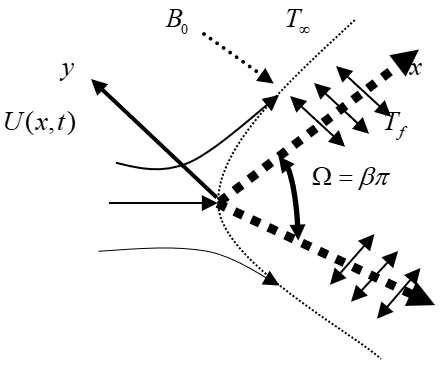Figure 1.  Flow configurations and coordinate system

Under the above assumptions and usual boundary layer approximation, the governing equations for this problem can be written as:

Continuity Equation:

$\frac{\partial u}{\partial x}\,+\,\frac{\partial v}{\partial y}\,=\,0$                         (1)

Momentum Equation:

$\frac{\partial u}{\partial t}\,+\,u\frac{\partial u}{\partial x}\,+v\frac{\partial v}{\partial y}\,=\,\frac{\partial U}{\partial t}\,+U\,\frac{\partial U}{\partial x}\,+{{\upsilon }_{a}}\,\frac{{{\partial }^{2}}u}{\partial {{y}^{2}}}\,$

$+\,\frac{S}{\rho }\,\frac{\partial N}{\partial y}\,-\frac{\sigma \,{{B}_{0}}^{2}}{{{\rho }_{\infty }}}(u-U)$                         (2)

Angular Momentum Equation:

$\frac{\partial N}{\partial t}\,+\,u\frac{\partial N}{\partial x}\,+v\frac{\partial N}{\partial y}\,=\frac{{{\upsilon }_{s}}}{\rho j}\,\frac{{{\partial }^{2}}N}{\partial {{y}^{2}}}\,-\,\frac{S}{\rho j}\,(2N+\,\frac{\partial u}{\partial y})$                (3)

Energy Equation:

$\frac{\partial T}{\partial t}\,+\,u\frac{\partial T}{\partial x}\,+v\frac{\partial T}{\partial y}\,=\frac{\kappa }{\rho {{c}_{p}}}\,\frac{{{\partial }^{2}}T}{\partial {{y}^{2}}}\,+\,\frac{{{Q}_{0}}}{\rho {{c}_{p}}}\,(T-\,{{T}_{\infty }})$             (4)

where $u$,$u$are the velocity components along $x$,$y$ co-ordinates respectively, $t$is the time, ${{\upsilon }_{a}}\,=\frac{\pi \,+\,S}{\rho }$ is the apparent kinematic viscosity, ${{\upsilon }_{s}}\,=\left( \mu \,+\frac{S}{\rho } \right)\,j$ is the microrotation viscosity (or spin-gradient viscosity), $\mu$  is the coefficient of dynamic viscosity, $S$ is the microrotation coupling coefficient (or vortex viscosity), $\rho$is the density of the fluid,  $N$is the microrotation component normal to the $xy$-plane,$j$is the micro-inertia density, $T$ is the temperature of the fluid within the boundary layer, ${{T}_{\infty }}$  is the free stream temperature, ${{c}_{p}}$ is the specific heat of the fluid at constant pressure, $\kappa$is the thermal conductivity and  is the heat generation/absorption constant.

2.2 Boundary conditions

The boundary conditions for the above problem are

(i) On the surface of the wedge (y = 0):

$u=\lambda U(\mathrm{x}, \mathrm{t}), \mathrm{v}=\pm \mathrm{v}_{w}(\mathrm{x}), \mathrm{N}=-\mathrm{n} \frac{\partial u}{\partial y},-\kappa \frac{\partial T}{\partial y}=h_{f}\left(T_{f}-T\right)$            (5a)

(ii) Matching with the free stream (y$\rightarrow$∞):

$u=U(\mathrm{x}, \mathrm{t})=\frac{v x^{m}}{\delta^{m+1}}, N=0, T=T_{\infty}$                       (5b)

where ${{v}_{w}}(x,t)$ represents the suction/injection velocity at the porous wedge where its sign indicates suction $(x<0)$ or injection $(x>0)$ and $U(x,t)$ is the potential velocity generated by the pressure gradient. The constant $\lambda >0$ corresponds to a stretching wedge and $\lambda <0$for a shrinking wedge while $\lambda =0$for a static wedge. The value of microrotation parameter $n=0$results $N=0$which represents no-spin condition i.e. the microelement in concentrated particle flow-close to the wall are not able to rotate as stated by Jena and Mathur . The case $n=0.5$ physically represents the vanishing of the anti-symmetric part of the stress tensor and represents weak concentrations of the micro-elements of the micropolar fluid at the solid surface. For this case Ahmadi  suggested that in a fine particle suspension the particle spin is equal to the fluid velocity at the wall.

2.3 Non-dimensionalization

To obtain similarity solutions of the governing equations (1)-(4) under the boundary conditions (5a) and (5b) we introduce the following non-dimensional variables:

$\eta=y \sqrt{\frac{m+1}{2}} \sqrt{\frac{x^{m-1}}{\delta^{m+1}}}, \psi=v \sqrt{\frac{2}{m+1}} \sqrt{\frac{x^{m+1}}{\delta^{m+1}}} f(\eta)$,

$\theta(\eta)=\frac{T-T_{\infty}}{T_{f}-T_{\infty}}, N=v \sqrt{\frac{m+1}{2}} \frac{x^{\frac{3 m-1}{2}}}{\delta^{\frac{3 m+3}{2}}} g(\eta)$          (6)

where $\eta$ is the similarity variable and $\psi$is the stream function  that satisfies the continuity equation (1) such that $u=\frac{\partial \psi }{\partial y}$ and $v=\,-\frac{\partial \psi }{\partial x}$.

Now substituting (6) into equations (2)-(4) we obtain:

$(1+\Delta) f^{\prime \prime \prime}+f f^{\prime}+\frac{2 m}{m+1}\left(1-f^{\prime 2}\right)+\Delta g^{\prime}$

$-K\left[2-2 f^{\prime}-\eta f^{\prime \prime}\right]-\frac{2 M}{m+1}\left(f^{\prime}-1\right)=0$          (7)

$b g^{\prime \prime}-\frac{2 \Delta B}{m+1}\left(f^{\prime \prime}+2 g\right)+f g^{\prime}-\frac{3 m-1}{m+1} f^{\prime} g+K\left(3 g+\eta g^{\prime}\right)=0$          (8)

$\theta^{\prime \prime}+\operatorname{Pr}(K \eta+f) \theta^{\prime}+\operatorname{Pr} Q \theta=0$          (9)

where$Q=\,\frac{{{Q}_{0}}}{\rho \,{{c}_{p}}}\,\frac{2}{m+1}\,\frac{x}{U}$ is the heat generation/absorption parameter,$\Delta =\frac{S}{\rho \,\upsilon }$ is the vortex viscosity parameter, $K=\frac{{{\delta }^{m}}}{\upsilon \,{{x}^{m-1}}}\,\frac{d\delta }{dt}$is the unsteadiness parameter, $M=\frac{\sigma B_{0}^{2}}{\rho \upsilon }\,\frac{{{\delta }^{m+1}}}{{{x}^{m-1}}}$is the magnetic field parameter, $b=\frac{{{\upsilon }_{s}}}{\rho \upsilon j}$ is the spin gradient viscosity parameter, $B=\frac{{{\delta }^{m+1}}}{j\,{{x}^{m-1}}}$ is the local micro-inertia density parameter and $\Pr =\frac{\mu {{c}_{p}}}{\kappa }$ is the Prandtl number.

The corresponding boundary conditions (5a) and (5b) becomes

$f=f_{w}, f^{\prime}=\lambda, g=-n f^{\prime \prime}, \theta^{\prime}=B i \sqrt{\frac{2}{m+1}}(\theta-1)$ at $\eta=0$          (10a)

$f^{\prime}=1, \theta=0, g=0 \quad$ as $\eta \rightarrow \infty$          (10b)

where ${{f}_{w}}=\,\pm {{v}_{w}}\left( x,\,t \right)\,\sqrt{\frac{2}{m+1}}\,\frac{{{\delta }^{\frac{m+1}{2}}}}{\upsilon {{x}^{\frac{m-1}{2}}}}$   is the wall mass transfer coefficient which is positive $({{f}_{w}}>0)$for suction and negative $({{f}_{w}}<0)$for injection $Bi=\frac{{{h}_{f}}}{\kappa }{{(Re)}^{-\,\frac{1}{2}}}$is Biot number or, the surface convection parameter.

2.4 Important physical parameters

The parameters of engineering interest for the present problem are the local skin friction coefficient (rate of shear stress), local plate couple stress and local Nusselt number (rate of heat transfer) which are given in the following expressions:

$\frac{1}{2}{{C}_{{{f}_{x}}}}{{\operatorname{Re}}^{\frac{1}{2}}}=\sqrt{\frac{m+1}{2}}[1+(1-n)\Delta ]{f}''(0)$                   (11)

${{M}_{{{w}_{x}}}}=\frac{b(m+1){g}'(0)}{(1+\Delta )B}$                                   (12)

${{N}_{{{u}_{x}}}}{{\operatorname{Re}}^{-\,\frac{1}{2}}}=Bi(1-\theta (0))$                     (13)

3. Method of Solution

The  nonlinear ordinary differential equations (7)-(9) along with the corresponding boundary conditions (10a) and (10b) have been solved numerically by applying sixth order Runge-Kutta integration scheme together with Nachtsheim-Swigert  shooting iteration technique with $\lambda ,\,\,Q,\,M,\,{{f}_{w}},\,\,m,$$K,\,n,\,\,\Delta ,\,\,b,\,\,B,\,\,\Pr$and $Bi$as prescribed parameter. A step size of $\Delta \eta =0.001$  was selected to be satisfactory for a convergence criterion of ${{10}^{-6}}$ in all cases. The value of ${{\eta }_{\infty }}$was found to each iteration loop by the statement ${{\eta }_{\infty }}={{\eta }_{\infty }}+\,\Delta \eta$. The maximum value of ${{\eta }_{\infty }}$ to each group of parameters $\lambda ,\,Q,\,M,\,{{f}_{w}},\,m,\,K,\,n,\,\Delta ,\,b,\,B,\,\Pr$and $Bi$determined when the value of the unknown boundary conditions at $\eta =0$ does not change to successful loop with an error less than ${{10}^{-6}}$.

3.1 Code verification

To assess the accuracy of the present code, we calculated the values of $f(\eta )$, ${f}'(\eta )$ and ${f}''(\eta )$ for the Falkner-Skan boundary layer equation when$\Delta =m=K=M=0$for different values of $\eta$. From Table 1, we observe that the data produced by the present code and that of White  are in excellent agreement. This gives us confidence to use the present numerical method.

Table 1. Comparison of the present numerical results with White  for Falkner-Skan boundary layer flow when  $\Delta =m=K=M=0$

 $\eta$ $f(\eta )$ $f(\eta )$ ${f}'(\eta )$ ${f}'(\eta )$ ${f}''(\eta )$ ${f}''(\eta )$. Present work White  Present work White  Present work White  0 0.0000 0.0000 0.0000 0.0000 0.4696 0.4696 1 0.2330 0.2330 0.4606 0.4606 0.4344 0.4344 2 0.8869 0.8870 0.8173 0.8167 0.2557 0.2557 3 1.7957 1.7956 0.9691 0.9691 0.0677 0.0677 4 2.7840 2.7839 0.9978 0.9978 0.0069 0.0067 5 3.7834 3.7832 0.9999 0.9999 0.0003 0.0002
4. Numerical Results and Discussion

Numerical calculations have been carried out for different values of the physical parameters such as stretching/shrinking parameter $\lambda$, heat generation/absorption parameter $Q$, magnetic field parameter $M$, suction/injection parameter ${{f}_{w}}$, pressure gradient parameter $m$, unsteadiness parameter $K$, microrotation parameter $n$, vortex viscosity parameter $\Delta$, spin-gradient viscosity parameter $b$, micro-inertia density parameter $B$ and Biot number $Bi$ keeping Prandtl number $\Pr$ as fixed. Here we have considered human blood as the micropolar fluid. At $T=\text{310}\ \text{K}$(human body temperature); the value of $\rho =1.05\times {{10}^{3}}kg/{{m}^{3}}$,${{c}_{p}}=14.65\,J/kgK$, $\kappa =2.2\times {{10}^{-3}}J/msK$ and$\mu =3.2\times {{10}^{-3}}kg/ms$. Thus, the value of the Prandtl number becomes $\Pr =\frac{\mu {{c}_{p}}}{\kappa }=21.0$  (see also Chato  and Valvano et al. ). The default values of other parameters are chosen as $\Delta =2.0$, $K=0.5$, $m=0.2$ , $B=0.5$, $b=2.0$, $n=0.5$, $M=0.5$, ${{f}_{w}}=0.5$, $\lambda =0.2$, $Q=0.5$  and $Bi=0.5$.

The effects of the stretching parameter $\lambda (>0)$ on the dimensionless velocity, microrotation and temperature profiles within the boundary layer are shown in Figures 2(a)-(c) respectively.  It can be illustrated from these figures that both the fluid velocity and microrotation profiles increase with the increase of $\lambda (>0)$ whereas the temperature within the boundary layer decrease with the increase of $\lambda (>0)$. Figure 2(b) also shows that the dimensionless microrotation profiles remain negative. The behaviors of shrinking parameter $\lambda (<0)$ are just opposite to stretching parameter $\lambda (<0)$ which are shown in figures 3(a)-(c) respectively.

## 2a.png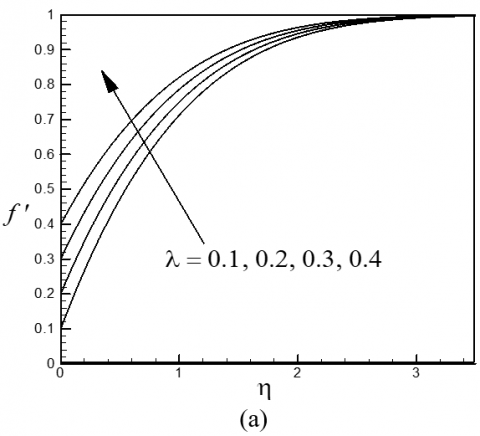## 2b.png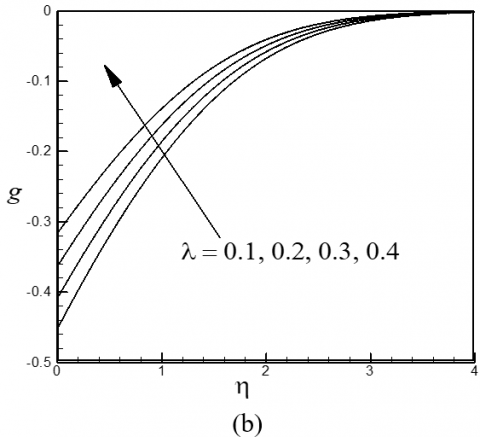## 2c.pngFigure 2. Variation of dimensionless (a) velocity, (b) microrotation and (c) temperature profiles for different values of $\lambda >0$

Figures 4(a)-(c) depict the dimensionless velocity, microrotation and temperature profiles for different values of the vortex viscosity parameter $\Delta$. From Figure 4(a) it can be seen that velocity profile decreases rapidly with the increases of $\Delta$ whereas the microrotation profiles increase with the increase of $\Delta$ shown in figure 4(b). The vortex visosity paraameter has narrow effect on temperature profiles .

The effects of the unsteadiness parameter $K$ on the dimensionless velocity, microrotation and temperature profiles are shown in Figure 5(a)-(c) respectively.

## 3a.png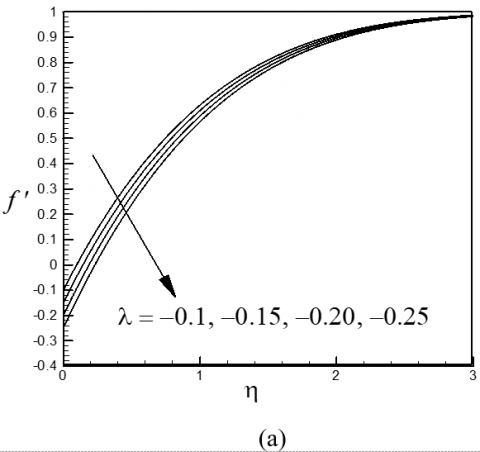## 3b.png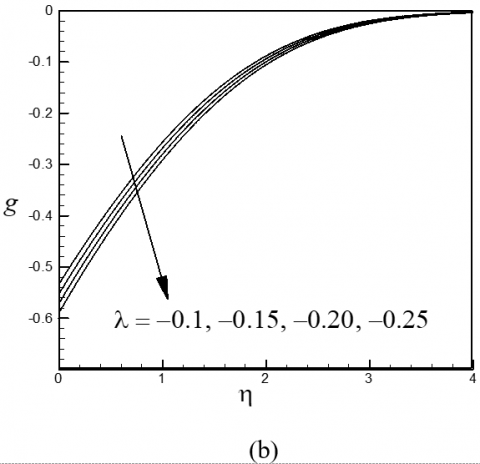## 3c.png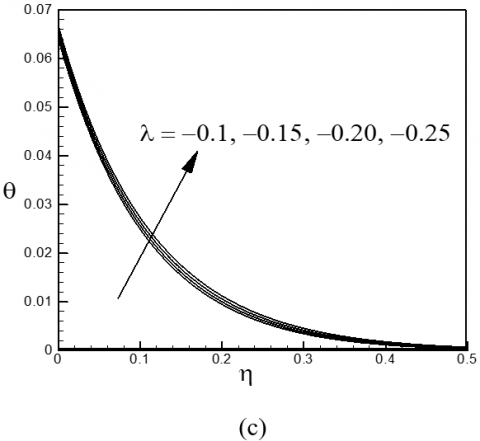Figure 3. Variation of dimensionless (a) velocity, (b) microrotation and (c) temperature profiles for different values of $\lambda <0$

From Figure 5(a), we observe that the velocity profiles decrease with the increasing values of $K$ for $\eta \le {{\eta }_{critical}}$. But for $\eta >{{\eta }_{critical}}$, a reverse trend is observed. This figure also  shows that a back flow phenomena occurs for $0<K<{{K}_{critical}}$ (not precisely determined). From Figure 5(b) we observe that microrotation of the blood corpuscles increases with the increase of $K$ in the vicinity of the surface of the wedge. But far away from the surface of the wedge where kinematic viscosity dominates the flow microrotation profiles overlap and decrease with the increase of $K$. Figure 5(c) shows that the non-dimensional temperature profiles within the boundary layer decrease with the increase of $K$. Therefore the unsteadiness parameter $K$ controls the flow, rotation of the micro-constituents and heat transfer characteristics.

## 4a.png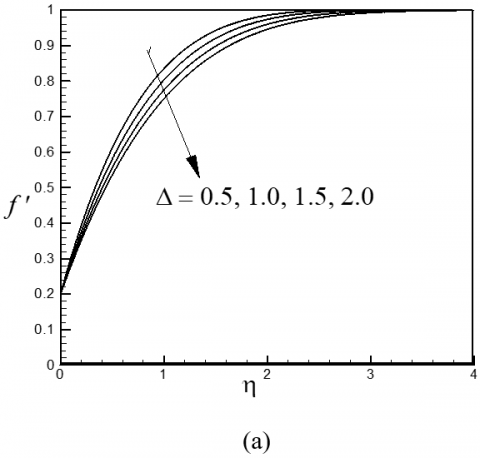## 4b.png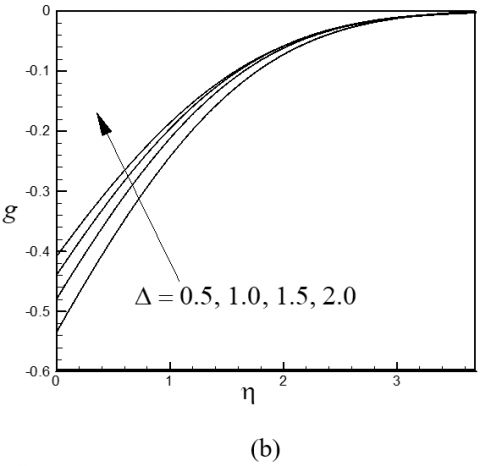## 4c.png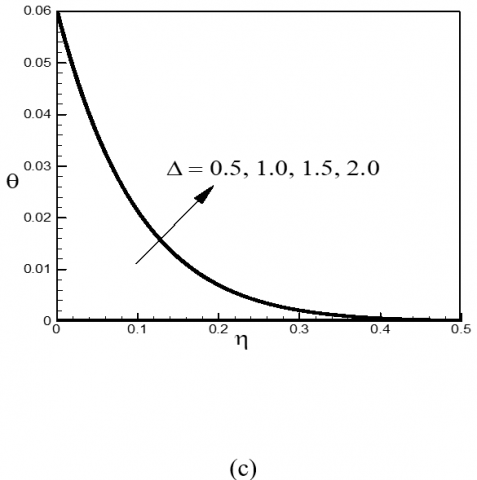Figure 4. Variation of dimensionless (a) velocity, (b) microrotation and (c) temperature profiles for different values of $\Delta$

Figures 6(a)-(c), respectively, depict the velocity, microrotation and temperature profiles for different values of the suction parameter ${{f}_{w}}>0$. From Figure 6(a) we observe that the fluid velocity inside the boundary layer increases with the increase of ${{f}_{w}}$. From Figure 6(b) it can be seen that, microrotation (angular velocity) of the microelements remains negative and decrease vary close to the surface of the wedge within the domain $\eta \le {{\eta }_{critical}}$  for increasing values of  ${{f}_{w}}>0$. But for $\eta >{{\eta }_{critical}}$this tendency is reversed in the upper portion of the boundary layer. Opposite phenomena occurs for both velocity and microrotation profiles for ${{f}_{w}}<0$which are shown in figures 7(a)-(b). The dimensionless temperature profiles decreases with the increase of both ${{f}_{w}}>0$ and ${{f}_{w}}<0$ which are shown in figures 6(c) and 7(c) respectively. Thus, decelerated fluid particles close to the heated wall absorb more heat from the surface of the wedge. When these decelerated fluid particles are sucked through the porous wedge leads to decrease of the fluid temperature. Thus, applying suction/injection, the growth of the hydrodynamic boundary layer thickness hence the speed of the flow and the temperature function can be controlled  that is important for many engineering applications like nuclear reactors, generators etc.

## 5a.png## 5b.png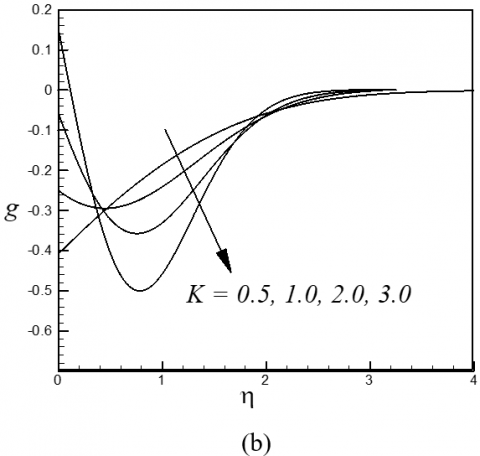## 5c.png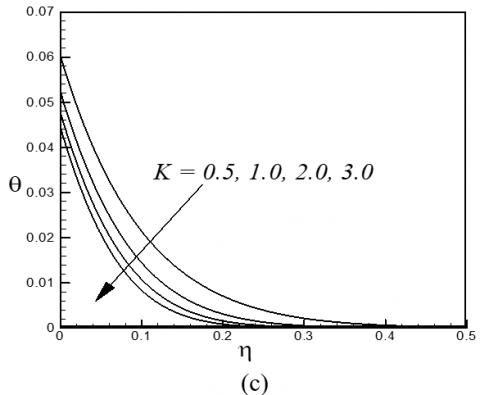Figure 5. Variation of dimensionless (a) velocity, (b) microrotation and (c) temperature profiles for different values of $K$

## 6a.png## 6b.png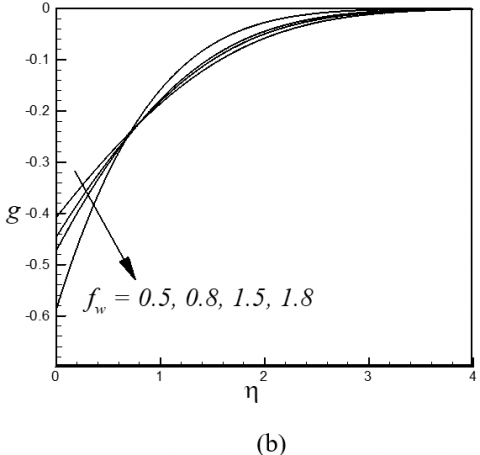## 6c.png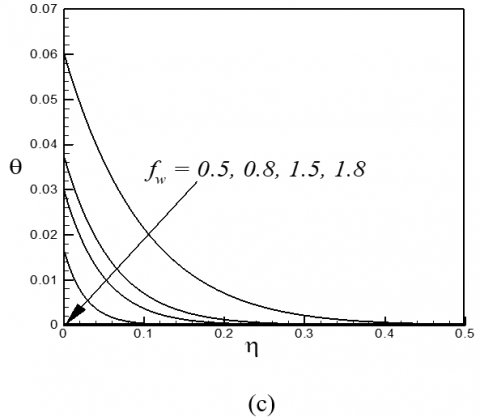Figure 6. Variation of dimensionless (a) velocity, (b) microrotation and (c) temperature profiles for different values of  ${{f}_{w}}>0$

Figures 8(a)-(b), respectively, show the non-dimensional velocity and microrotation profiles across the boundary layer for different values of $n$. The above-mentioned calculations have been done for a weakly concentrated micropolar fluid i.e. for the microrotation parameter $n=0.5$. Figure 8(a) reveals that as increases the concentration of the fluid decreases hence fluid velocity increases whereas microrotation profiles decrease with the increase of $n$shown in figure 8(b). The case $n=0$ corresponds to the boundary condition $g(0)=0$indicating the no-spin condition. From this figure we see that for $n=0$, the solution remains positive and increases from zero to zero as $\eta$ increases from zero to infinity. The case$n=0.5$ correspond to zero anti-symmetric part of the stress tensor. The effect of the Biot number (surface convection parameter) Bi on the temperature profiles against η are displayed in Figure 8(c). This figure illustrate the temperature profiles within the boundary layer increase with the increase of Bi. From this figure it is also confirmed that for large values of Bi i.e. Bi→∞, the temperature profile attains its maximum value 1: thus the convective boundary condition become the prescribed surface temperature case.

## 7a.png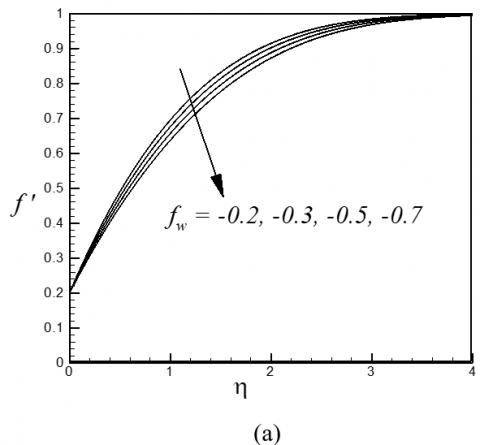## 7b.png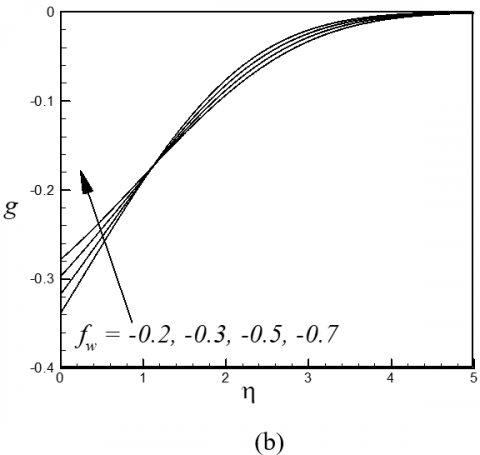## 7c.pngFigure 7. Variation of dimensionless (a) velocity, (b) microrotation and (c) temperature profiles for different values of  ${{f}_{w}}<0$

## 8a.png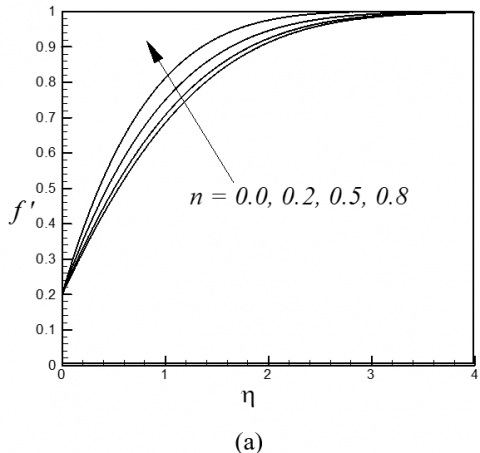## 8b.png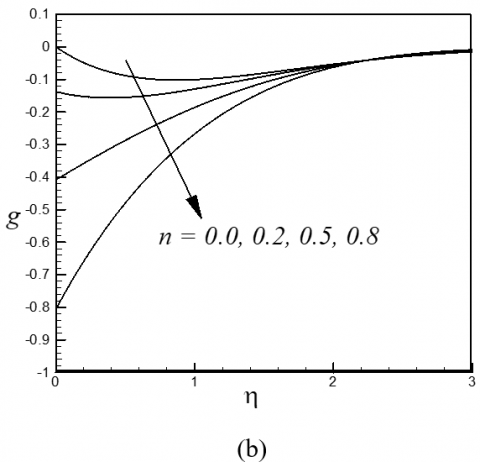## 8c.png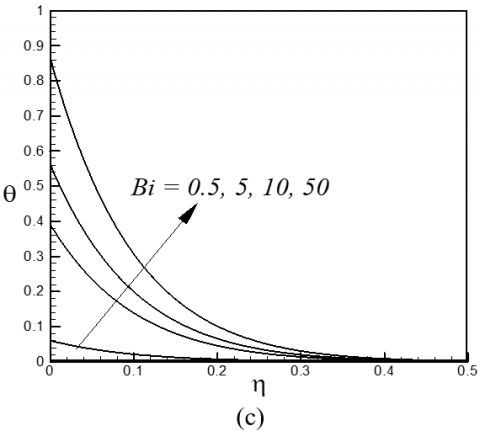Figure 8. Variation of dimensionless (a) velocity and (b) microrotation profiles for different values of $n$and (c) temperature profiles for different values of Bi

The effect of the heat generation/absorption  on the temperature profiles against $\eta$ are displayed in Figure 9. This figure illustrate the temperature profiles within the boundary layer increase with the increase of $Q$. It is also apparent that the thickness of the thermal boundary layer increase with the increase of heat generation.

## 9.png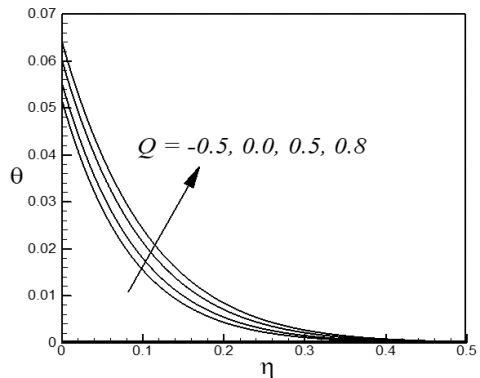Figure 9. Variation of dimensionless temperature profiles for different values of $Q$

Table-2 shows the local skin-friction coefficient, local plate couple stress and the local Nusselt number for different values of $\lambda$ , $Q$ and  keeping all other parameters fixed. From this table we observe that the local skin-friction coefficients (rate of share stress), the local plate couple stress and the local Nusselt number (rate of heat transfer) decrease with the increase of the magnitude of $\lambda \,(\lambda >0)$. Reverse phenomena is noticed for the case of $\lambda <0$which is not shown here. The both local skin-friction coefficients and the local plate couple stress with the increase of  $M$ whereas the local Nusselt number decrease with the increase of $M.$ Again, the local Nusselt number increase with the increase of $Q\,(Q>0)$. The heat generation mechanism increases the fluid temperature near the surface of the wedge as a consequences rate of heat transfer from the hot surface of the wedge to the cold fluid increase. Therefore, it may be beneficial in flow and temperature controls of polymer processing.

Table 2. Numerical values of the local skin-friction coefficient, plate couple stress and the local Nusselt number for different values of  λ, Q and M

 λ Q M f'' (0) g' (0) -θ' (0) 0.2 0.2 0.5 0.8168921 0.2581758 0.0603921 0.5 0.5348498 0.1754071 0.0575329 0.8 0.2331363 0.0757795 0.0552402 1.0 0.0000006 0.0000002 0.0526031 0.2 0.2 0.5 0.8168921 0.2581758 0.0603921 1.0 0.9655778 0.3402117 0.0602641 2.0 1.1995842 0.4759693 0.0600709 3.0 1.3874870 0.5897992 0.0599271 0.2 0.2 0.5 0.8168921 0.2581759 0.0572852 0.5 0.8168921 0.2581758 0.0603921 0.8 0.8168921 0.2581758 0.0642567 1.0 0.8168921 0.2581758 0.0792374
5. Conclusions

Unsteady hydromagnetic boundary layer heat transfer flow of a micropolar fluid over a forced convectively heated permeable linearly shrinking/stretching wedge with heat generation/absorption is investigated numerically. From the numerical c$Q$omputations the following major findings can be concluded:

• Local skin friction coefficient, plate couple stress and rate of heat transfer decreases with the increase of stretching parameter whereas opposite phenomena occurs for shrinking parameter.
• Dimensionless velocity at the surface of the wedge increase/decreases with the increase of both stretching/shrinking and suction/injection parameter.
• Dimensionless temperature increases with the increase of shrinking parameter whereas it decreases with the increase of stretching parameter, unsteadiness parameter and suction/injection parameter.
• Near the wedge surface, angular velocity increase as unsteadiness parameter increases but opposite trend is observed far away from the wedge surface.
• Magnetic field increase skin friction coefficient and plate couples stress whereas it decreases heat transfer rate.
• Both the heat generation parameter and surface convection parameter increase temperature.
References

 Eringen, A. C., “Theory of micropolar fluids,” J. Math. Mech., vol. 16, pp. 1-18, 1966. DOI: 10.1512/iumj.1967.16.16001.

 Eringen, A. C., “Theory of thermomicropolar fluids,” J. Math. Anal. Appl., vol. 38, pp. 480-496, 1972. DOI: 10.1016/0022-247X(72)90106-0.

 Crane, L. J., “Flow past a stretching plate,” J. Appl. Math. Phys. (ZAMP), vol. 21, pp. 645-647, 1970. DOI: 10.1007/BF01587695.

 Miklavčič, M. and Wang, C.Y., “Viscous flow due to a shrinking sheet,” Quart.Appl. Math., vol. 64, pp. 283-290, 2006.

 Ariman, T., Turk, M. A. and Sylvester, N. D., “Microcontinuum fluids mechanics-a review,” Int. J.  Engng. Sci., vol. 11, pp. 905-930, 1973. DOI: 10.1016/0020-7225(73)90038-4.

 Ariman, T., Turk, M. A. and Sylvester, N. D., “Applications of microcontinuum fluids mechanics,” Int. J. Engng. Sci., vol. 12, pp. 273-293, 1974. DOI: 10.1016/0020-7225(74)90059-7.

 Soundalgeker, V. M. and Takhar, H. S., “Flow of a micropolar fluid on a continuous moving plate,” Int. J. Engg. Sci., vol. 21, pp. 961-970, 1983.

 El-Arabawy, H.A.M., “Effect of suction/injection on the flow of a micropolar fluid part a continuously moving plate in the presence of radiation,” Int. J. Heat Mass Tran., vol. 46, pp. 1471-1477, 2003. DOI: 10.1016/S0017-9310(02)00320-4.

 Alam, M. S., Sattar, M. A., Rahman, M. M. and Postelnicu, A., “Local similarity solution of an unsteady two-dimensional MHD convective flow of a micropolar fluid past a continuously moving porous plate under the influence of magnetic field,” Int. J. Heat & Tech., vol. 28(2), pp. 95-105, 2010.   http://www.iieta.org/sites/default/files/Journals/HTECH/.

 Rahman, M. M., Eltayeb, I. A. and Rahman, S. M. M., “Thermo-micropolar fluid flow along a vertical permeable plate with uniform surface heat flux in the presence of heat generation,” Thermal Sci., vol. 13, pp. 23-36, 2009.   DOI: 10.2298/TSCI0901023R.

 Rahman, M. M., and Sattar, M. A., “Magnetohydrodynamic convective flow of a micropolar fluid past a continuously moving vertical porous plate in the presence of heat generation/absorption,” ASME J. Heat Trans., vol. 128, pp. 142-152, 2006. DOI: 10.1115/1.2136918.

 Jena, S. K. and Mathur, M. N., “Similarity solution for laminar free convection flow of thermo-micropolar fluid past a non-isothermal vertical flat plate,” Int. J. Eng. Sci., vol. 19, pp. 1431-1439, 1981. DOI: 10.1016/0020-7225(81)90040-9.

 Ahmadi, G., “Self similar solution of incompressible micropolar boundary layer flow over a semi-infinite plate,” Int. J. Eng. Sci., vol. 14, pp. 639-646, 1976. DOI: 10.1016/0020-7225(76)90006-9.

 Nachtsheim, P. R. and Swigert, P., “Satisfaction of the asymptotic boundary conditions in numerical solution of the system of non-linear equations of boundary layer type,” NASA TND-3004, 1965. http://ntrs.nasa.gov/archive/nasa/casi.ntrs.nasa.gov/19650026350.pdf

 F. M. White, “Viscous Fluid Flows,” Third Edition, McGraw-Hill, New York, 2006.

 Chato, J. C., “Heat transfer to blood vessels,” J. Biomech Eng., vol. 102 (2), pp.110-118, 1980.       DOI: 10.1115/1.3138205.

 Valvano, J. W., Nho, S. and Anderson, G. T., “Analysis of the Weibaum-Jiji model of blood flow in the canine kidney cortex for self-heated thermistors,” J. Biomech Eng., vol. 116 (2), pp.201-207, 1994. DOI: 10.1115/1.2895720.